﻿ Getting Started Tutorials - MechDesigner > Tutorial 18: Math FB > Step 18.1: Position: Plot an Epitrochoid Curve

# Step 18.1: Position: Plot an Epitrochoid Curve

### Plotting the Epitrochoid-Curve: Position Equations

In this step, we enter in the Math FB the parametric equations that define the X and Y coordinates for the shape of the Epitrochoid-Curve.

The Epitrochoid-Curve is a good example. We can compare the Epitrochoid Curve derived from the Math FB with the curve we can get from a Gear-Pair.

The Epitrochoid-Curve given by a Gear-Pair will be correct - it is found by MechDesigner!

The Epitrochoid-Curve from the Math FB will only be correct when our equations are correct.

See Tutorial 14 : Epitrochoid Curve given by a Gear-Pair.

#### Parameters and Parametric Equations

 We will add parametric equations to a Math FB to calculate separately the X and Y coordinates of the Epitrochoid-Curve. One equation will calculate the X–axis coordinates. A different equation will calculate the Y–axis coordinates.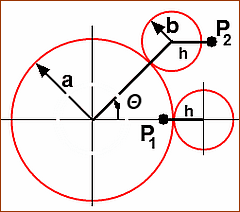The parametric equations for the family of epitrochoid curves are: Px = (a+b)*cos(Θ) – h*cos((a+b)/b)*Θ) Py = (a+b)*sin(Θ) - h*sin((a+b)/b)*Θ) Parametric-Constants a = radius of the fixed circle b = radius of the rolling circle h = distance to the point, P, from the center of the rolling circle Independent-Variable Θ = the independent variable: 0 to 360 (0 or 2×pi) The parametric equations, above, give the family of Epitrochoid Curves. Thus, if you change a Parametric-Constant - a, b, h - you will plot a different Epitrochoid-Curve. There are two procedures to enter different the parametric-constants in the Math FB. Procedure 1: Enter the actual values for the Parametric-Constant - a, b, h - explicitly in the Math FB. For example, enter the values 110, 20, 14 for each constant. To plot a different Epitrochoid Curve, we open the Math FB dialog-box to edit the parameters. Procedure 2: Specify a constant in a Gearing FB as the input to the Math FB To plot a different Epitrochoid Curve, edit the Gearing FB to change a parametric-constant. Or, even more efficient, add the parameters in the Gearing FB to a Design-Set.

#### Piggyback Sliders and Parametric Equations.The Mechanical model in MechDesigner

Because we will use Parametric Equations, it is very convenient to use the standard model of Piggyback Sliders.

 1.Edit the Base-Part, to add a horizontal Line; Exit the Part-Editor 2.Add the X-Sliderto move parallel with the X-axis, Do: Add a Part; Add a Slide-Joint between the Part and the horizontal Line in the Base-Part; Add a Motion-Dimension FB to the Sliding-Part to make it the X-Slider. 3.Add a vertical Line to the X-Slider. Do: Edit the X-Slider; Add a vertical Line that is parallel to the Y-axis; Exit the Part-Editor. 4.Add the Y-Slider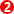to move parallel with the Y-axis of of the Base-Part. Do Add a Part; Add a Slide-Joint between the Part and the vertical Line in the X-Slider; Add a Motion-Dimension FB to the Sliding-Part to make it the Y-Slider. 5.Rename the two Motion-Dimensions to X-axis and Y-axis, as appropriate.

The Piggyback Sliders are now in the graphic-area.

#### Add a Math FB and Open the Math FB dialog-box1.Click Modeling FB toolbar > Add  Math FB 2.Click the graphic-area

The Math FB is now in the graphic-area.

Open the Math FB dialog-box

 1.Double-click the Math FB - or - 1.

The Math FB dialog-box is now open.

#### How many Input-Connectors and Output-Connectors?Input-Connectors

There are three(3) Parametric-Constants (a, b, h), plus the Independent Variable (Θ)

 1.Click the Add Input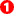button four(4) times to add four(4) input-connectors 2.Click the Updatebutton

Output-Connectors

We need a total of two(2) output-connectors as we will calculate parametrically the motion of the X-axis Slider and the Y-axis Slider

There is one output-connector when we add the Math FB to the graphic-area.

STEP 2: Add one(1) output-connector (a total of two(2) output-connectors):

 1.Click the Add Output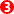button one(1) 2.Click the Updatebutton

Output Data Type

The Units at the output-connectors from the Math FB are set with the Output Data Type.

STEP 3: Change the Output Data Type to Linear Coordinates:

 1.Click the Output Data Type drop-down handle Drag the right of the Math FB dialog-box to see the Output Data Type drop-down handle. 2.Select Linear Coordinates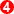- at the top of the list. 3.Click the Update buttonDefault Equations and Data-Channels and Output-Connectors

Note - See image above for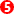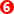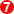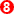# of Equations = 3 × # of Output-Connectors 3 Equations ×2 Output-connectors = Equations: 0 - 5Data-Channels = 3 for each Output-Connector •Dis - Linear Displacement, m •Vel - Linear Velocity - m/s •Acc - Linear Acceleration, m/s/s The units are always SI unitsThe default equations for the output-connectors 0 and 1 are: p(0),v(0),a(0) - three(3) Data-Channels connected to input-connector 0

#### POSITION Parametric Equations in the Math FB

The parametric equations for the family of Epitrochoid Curves are:

Px = (a+b)*cos(Θ) - h*cos((a+b)/b)*Θ)

Py = (a+b)*sin(Θ) - h*sin((a+b)/b)*Θ)

We must re-write these equation with the correct syntax for the Math FB.

Each of the four(4) parameter we connect to an input-connector has a wire-number (0, 1, 2, 3) and a data-channel (p, v a).

We use the p data-channel for the parameters. The v data-channel of a constant is equal to zero(0). Therefore, in the parametric equations we:

STEP 1: Enter the Parametric Equations for the two output-connectors

 Replace: a with p(0) ; b with p(1) ; h with p(2) ; Θ with p(3) 1.Equation #0 is now ... (p(0)+p(1))*cos(p(3))-(p(2)*cos(((p(0)+p(1))/p(1))*p(3))) 2.Click the Update button to confirm the changes 3.Equation #3 is now ... (p(0)+p(1))*sin(p(3))-(p(2)*sin(((p(0)+p(1))/p(1))*p(3))) 4.Click the Update button to confirm changes.

The equations in the Math FB are now: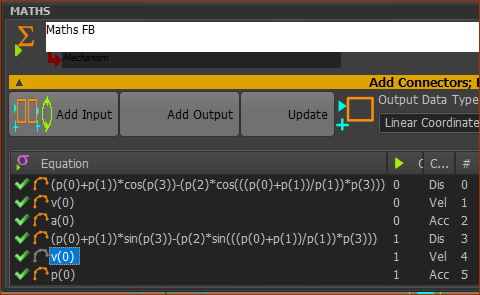#### Function-Blocks for the Parametric-Constant and the Independent Variable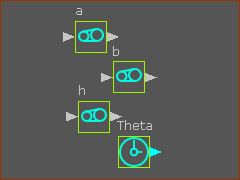Define the 3 Parametric-Constants

 1.Mechanism-Editor: Click Kinematic FB toolbar >  Add Gearing FBs - 3 x 2.Mechanism-Editor: Rename each Gearing FB to a, b, and h - see image to the left. 3.Mechanism-Editor: Edit each Gearing FB 'a' 4.Gearing FB dialog-box: Enter a value for the Add after Gearing Ratio parameter, 5.Close the Gearing-FB Do 3-5 again and again to enter values also for the Parametric-Constant b, and h.

Note: I have entered a = 120, b = 40, h = 40

<<< Gearing FB dialog-box : Add after Gearing Ratio for the Parametric-Constant 'a'

 1.Mechanism-Editor: Click Kinematic FB toolbar >Add Linear Motion FB The output from a Linear Motion FB increases from 0 to 360 and it is . It will represent the angle, Θ, the Independent-Variable,   See schematic image at the very top of this topic.

Connect the FBs

 1.Mechanism-Editor: Drag wires from the output of Gearing FB to the respective input-connectors of the Math FB 2.Mechanism-Editor: Drag a wire from the output of the Linear-Motion FB to the bottom input-connector of the Math FB. 3.Mechanism-Editor: Drag a wire from the Math FB for the X-axis to the input-connector of the Motion-Dimension FB for the Slider that will move parallel with he X-axis 4.Mechanism-Editor: Drag a wire from the Math FB for the Y-axis to the input-connector of the Motion-Dimension FB for the Slider that will move parallel with he Y-axis

 1.Mechanism-Editor: Click Kinematic elements toolbar > Add Trace-Point 2.Mechanism-Editor: Click a Point on Y-Slider of the Piggyback Slider 3.Mechanism-Editor: Click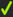in the Command-Manager.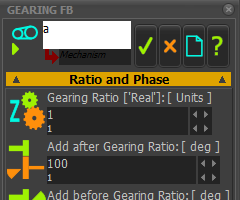Gearing FB: Add after Gearing Ratio parameter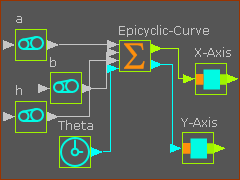#### Add a Design-Set - select the 3 Parametric-Constants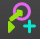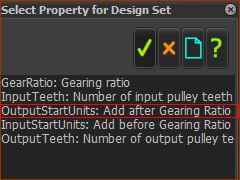1.Mechanism-Editor: Click Modeling elements toolbar > Add Design-Set , and click the graphic-area. 2.Mechanism-Editor: Open the Design-Set dialog-box 3.Design-Set dialog: Add three Element Rows, select one Element-Row 4.Design-Set dialog: Click a Gearing FB - for Parametric-Constant a Select Property for Design-Set selection interface opens - see image to the left. 5.Select Property for Design-Set : Select OutputStartUnits: Add after Gearing Ratio 6.Select Property for Design-Set: Clickto close the Select Property for Design-Set interface. Do 4 -6 again, and again, to add Parametric-Constants b and hEpitrcohoid Curve - Position Equations Only When we choose 120 for Parameter a, we can enter 60, 40, 30, 20, 10 as factors for Parameter b. Each will give a continuous, endless, Epitrochoid-Curve. •If Parameter h > b, there is a loop in the Epitrochoid-Curve.